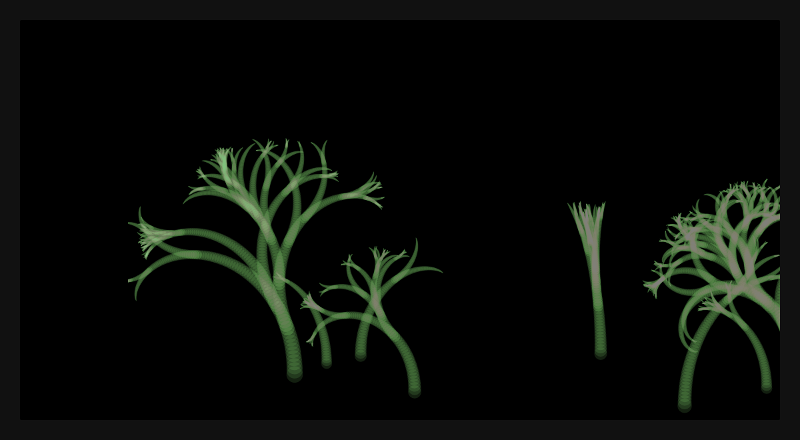## ronin experiment 9 - ghost trees

I created some translucent recursive trees that fade away to the right using roninThis is the recursive weirdness that leads to the animation above

``````(defn rec (v d) (
(if (gt v 1) (
(fill
(circle 0 0 (div v 10))
"#A0FF801F")
(pushTransform)
(move (div v 20) 0)
(rotate d)
(rec (sub v 1) d)
(popTransform)
(if (eq (floor (random 20)) 0) (
(pushTransform)
(rec (sub v 1) (sub 0 d))
(popTransform)
))
))))

(resetTransform)
(clear)

(on "animate" '(

(resetTransform)
(move (div (of (frame) :c) 200) 0)
;(scale 0.99)
(translate (frame) (pos 0 0))
(resetTransform)
(fill (rect 0 0 3 (of (frame) :h) ) "black")

(fill (frame) "#00000001")
(if (eq (floor (random 50)) 0) (
(move 250 (random (sub (of (frame) :h) 100) (of (frame) :h)))
(pushTransform)
(rotate (div PI -2))
(rec (random 80 130) (random -0.05 0.05))
(popTransform)
))
(resetTransform)
))
``````